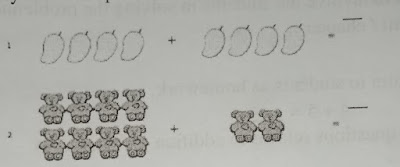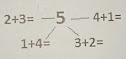Lesson Planning of Number Operations (Addition)

Subject Mathematics

Students` Learning Outcomes

• Add two one digit numbers (sum up to 9)

Information for Teachers

• Addition means to take two sets of objects and combine them.
• While teaching the lesson, the teacher should also consult textbook at all steps wherein and whenever it I applicable.

Material / Resources

Number flashcards, stone/pebbles, writing board, chalk/marker, duster

Introduction

• Ask students what symbols should be place between these numbers
• Write plus ‘+’ on the board and ask students to name the sign. Write equality sign ‘=’ and ask same question.

Development

 Activity 2 Show some objects to students as given or draw on the board. Ask students to count number of mangoes in one group and write on their copies and then count the number of mangoes in the other group. Ask them to combine both groups and count again to find sum of both numbers. Repeat activity for other pictures.Activity 3 Show some fingers of one hand to students and ask them to write the numbers in their notebooks then show fingers from the other hand and ask them to write it in their notebooks as well.Ask them to write an equation for the number of fingers you have shown and their total. Now, ask how many fingers were shows in total. Write 5+3 on the board. Ask students to use their fingers of both hands to show numbers written on board then combine to find the sum. Repeat this activity for other numbers, keeping in mind that the sum should be less than 9.

 Activity 4 Give the groups 9 blocks each. Ask them to count 2 blocks. Now add three more blocks. Write an addition equation on paper  2+3=  □How many total blocks are there? Tell them that to make up different arrangements with the blocks and write the equations.

 Activity 5 Write number 5 on the board and ask student to find all those pair of numbers whose sum is 5. Write the responses on board. Repeat this activity for 6 to 9 in groups. Ask students to record the work during activity on their notebooks.

Sum up / Conclusion

Conclude that:

• Addition means combining the number of items of two groups.

Assessment

• Write some addition equations on the writing board and ask two students to solve.
• Give a bag containing number of flashcards ( 1 to 4) and ask two students to draw two cards.
• Ask them to write the equation and find the sum.
• Teacher is also required to involve the students in solving the problems given in the exercise at the end of unit/chapter.

1.